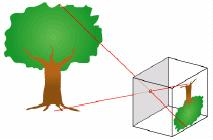# Similar Triangles

The current article deals with a frequently occurring topic in geometry in CAT- similarity of triangles. Most of you will notice that this topic is coming year after year in CAT and, as geometry questions in CAT are easy compared with other topics, mastering the concept is easier than mastering concepts in Algebra, Permutation, and Combination, or Probability.Similar triangles present one of the biggest tools students have to solve many geometry questions. And yet, whenever the opportunity arises, the students often fail to spot the similarity between two triangles. Sometime they spot the similarity but fail to apply the ratios correctly. Today we will start with some familiar cases in which we apply similarity of triangles and then move on to some tricky and difficult cases. We hope that after solving all these problems, you’ll not have any issue in spotting and solving similarity.

In practical scenario, we always prove that two triangles are similar by proving that their corresponding angles are equal. For example, look at the very simple figure below:If you can prove that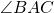=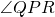and=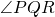then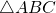and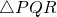are similar. In which case the ratios of the corresponding sides are equal. The best way to write the ratio of the sides is to write both the triangles in the order of the angle which are equal (for example here=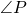,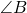=and=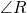so write ABC and PQR only) and then write the ratio by picking same corresponding points from the two written triangles. Therefore,